# RD Sharma Solutions for Class 9 Maths Chapter 20 Surface Area and Volume of A Right Circular Cone

## RD Sharma Solutions Class 9 Maths Chapter 20 – Free PDF Download

RD Sharma Solutions for Class 9 Maths Chapter 20 Surface Area and Volume of A Right Circular Cone comes with questions and answers related to the cone and its dimensions. The subject experts at BYJU’S outline the concepts in a clear and precise manner based on the IQ level of students. Each and every answer to all questions in RD Sharma Solutions are given here in a detailed manner to help the students understand the topics more effectively. This helps students to get a good score in the examinations along with providing extensive knowledge about the subject.

Students can make use of  RD Sharma Solutions for Class 9 to grasp the concepts in a precise manner and secure high marks in academics. Thorough practice of textbook problems with the help of RD Sharma Solutions clears doubts quickly and boosts conceptual knowledge among students. Students can download the solutions in PDF format from the links given below.

## Download PDF of RD Sharma Solutions for Class 9 Maths Chapter 20 Surface Area and Volume of A Right Circular Cone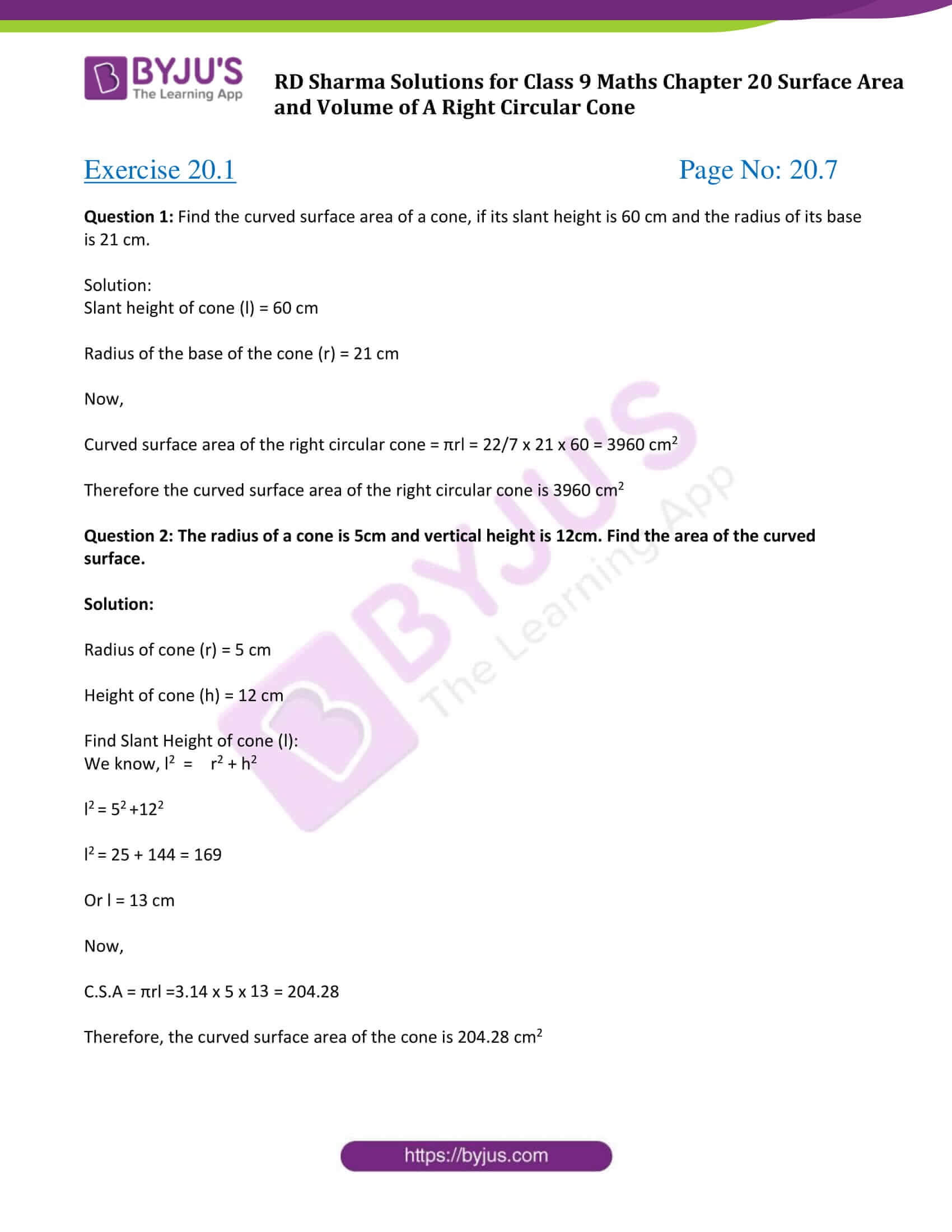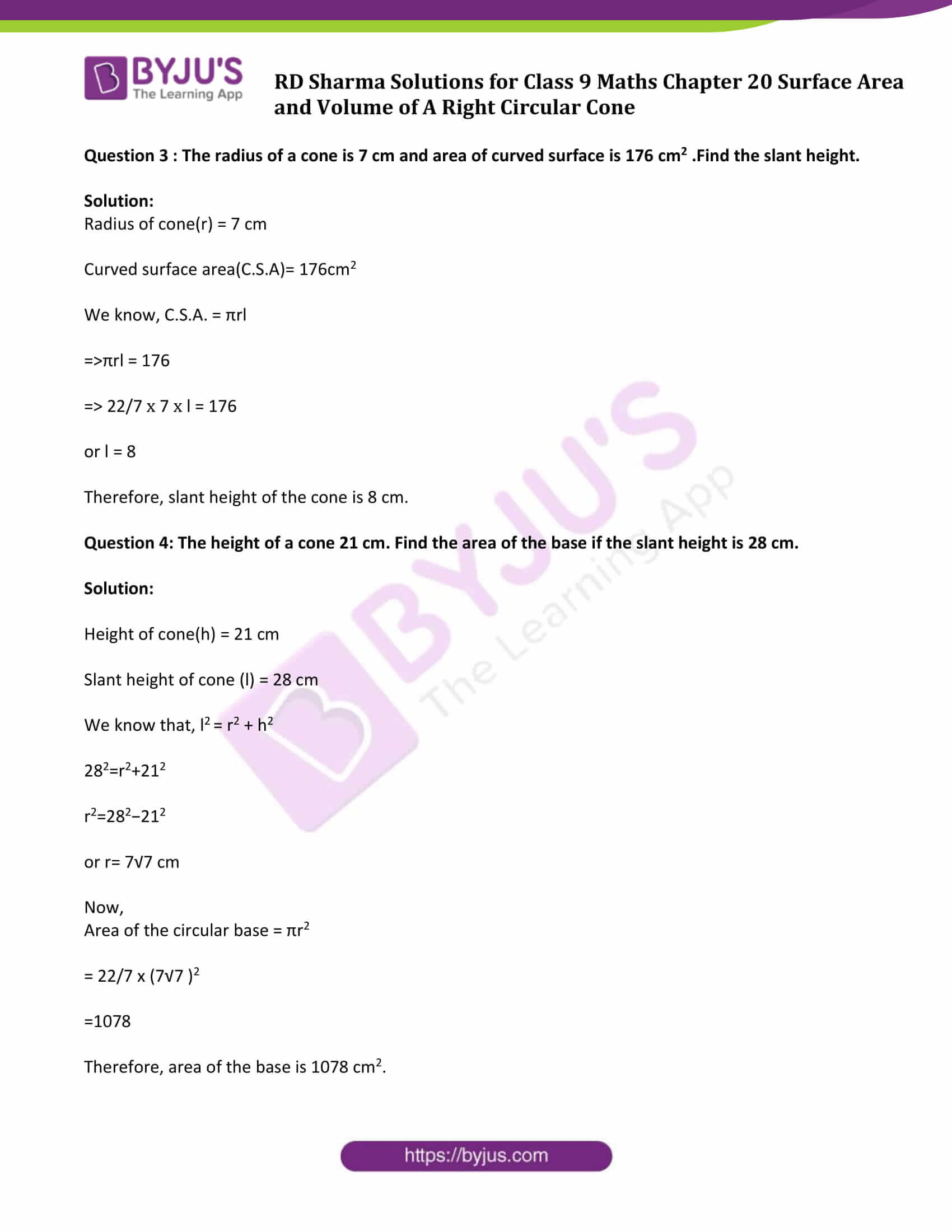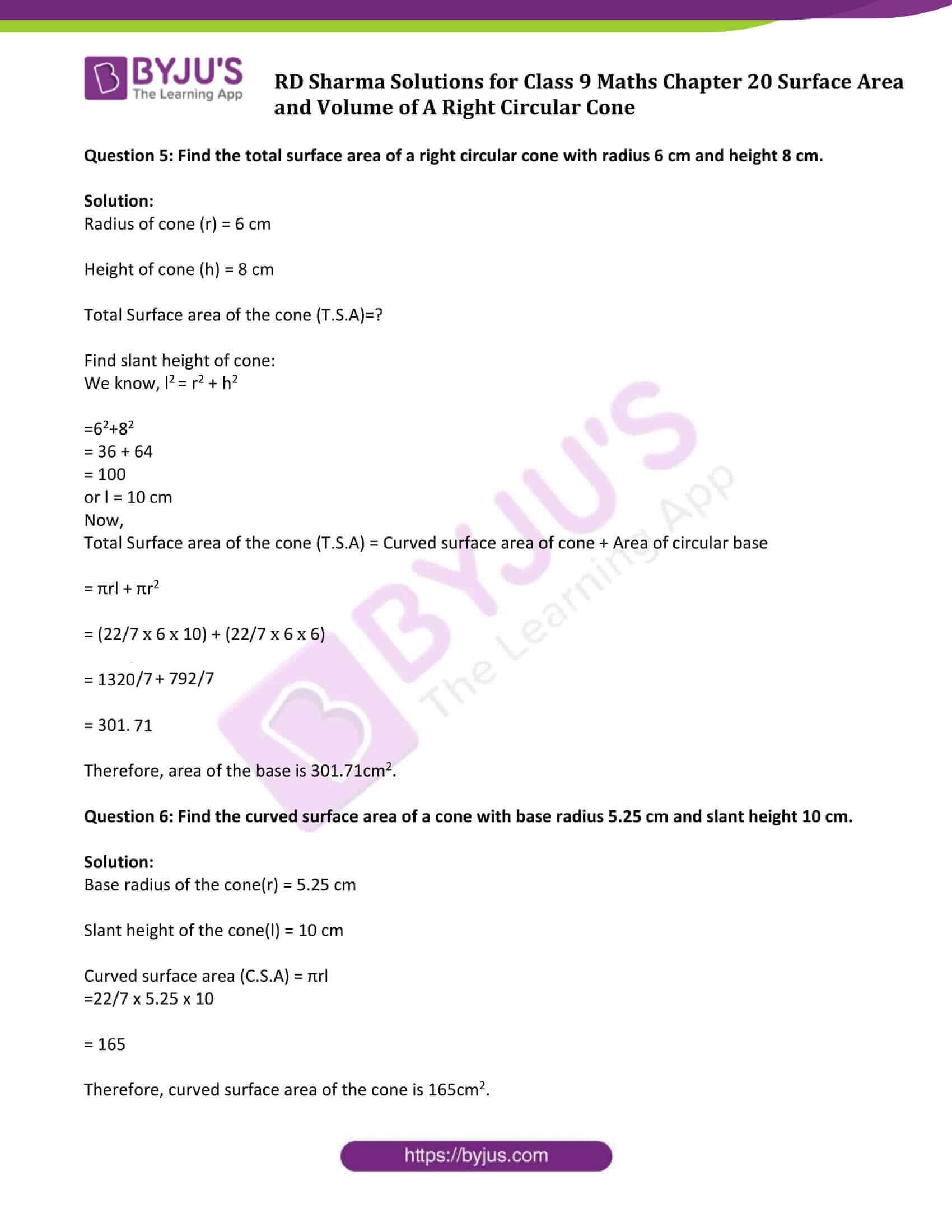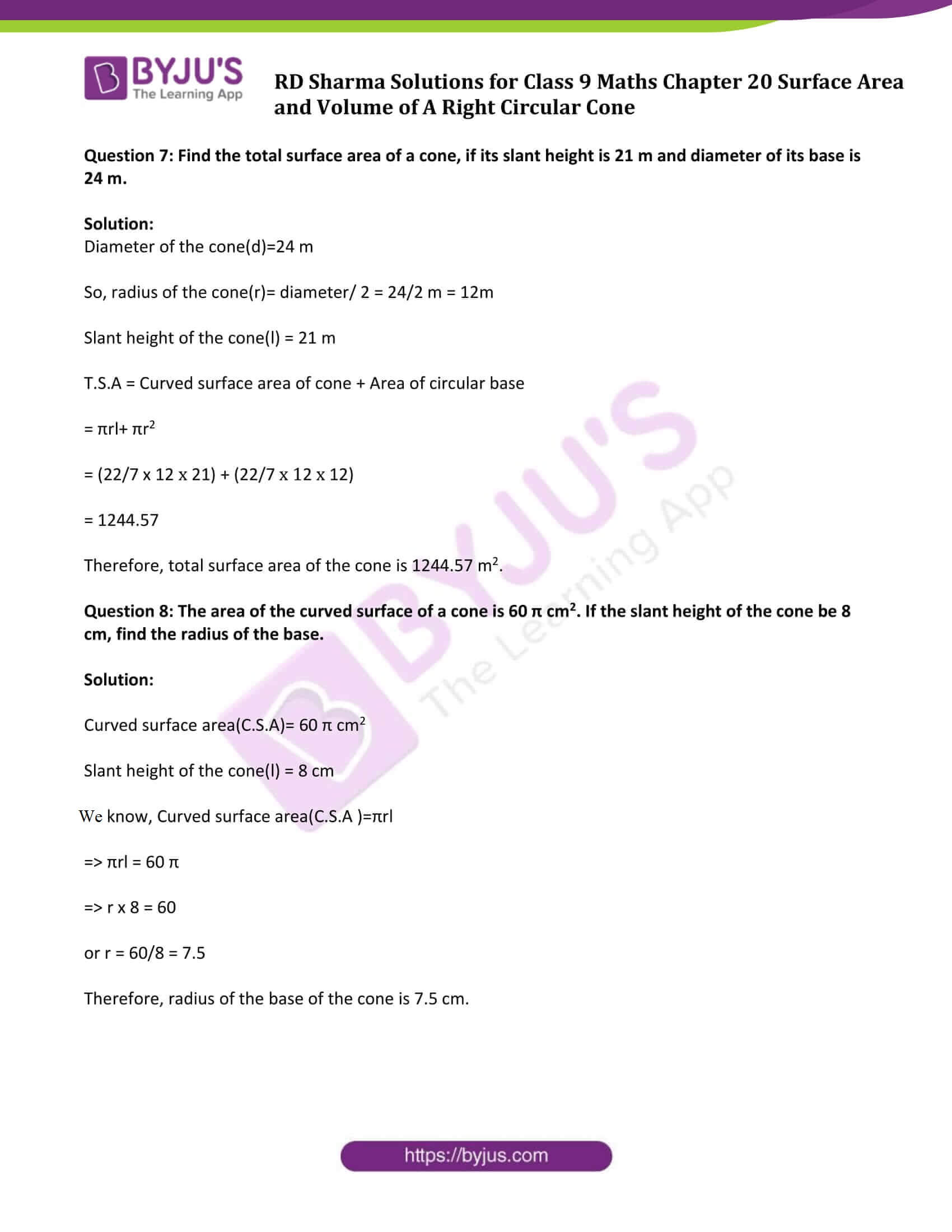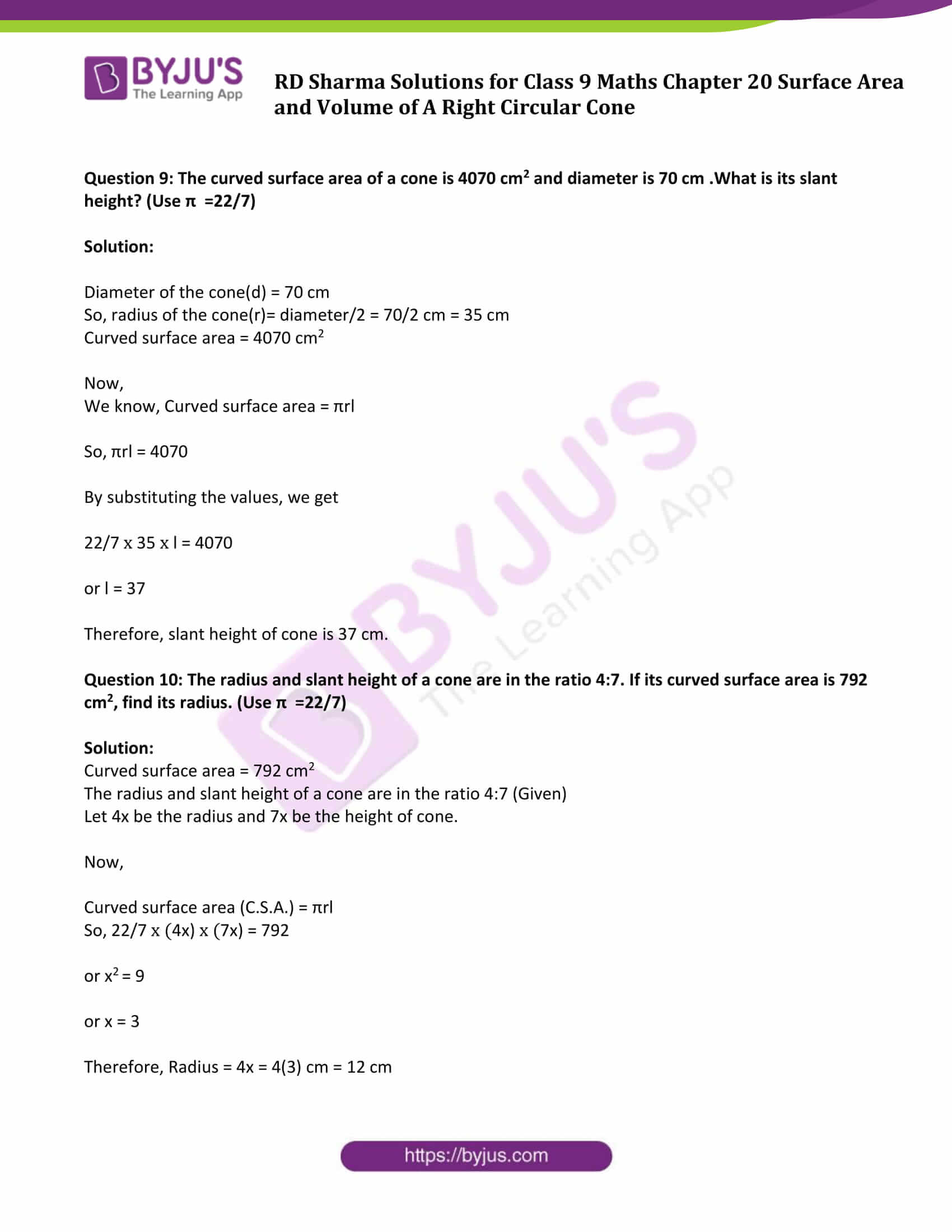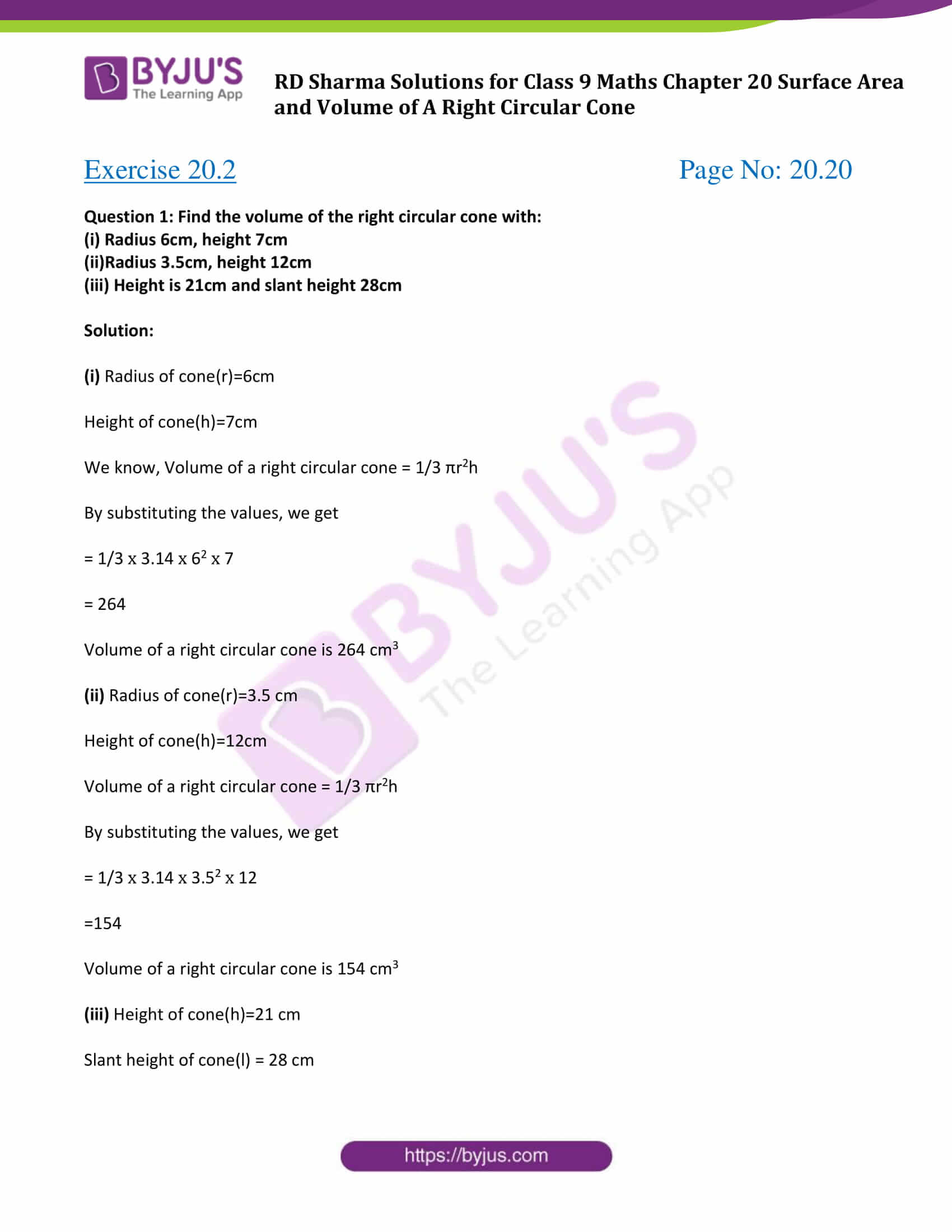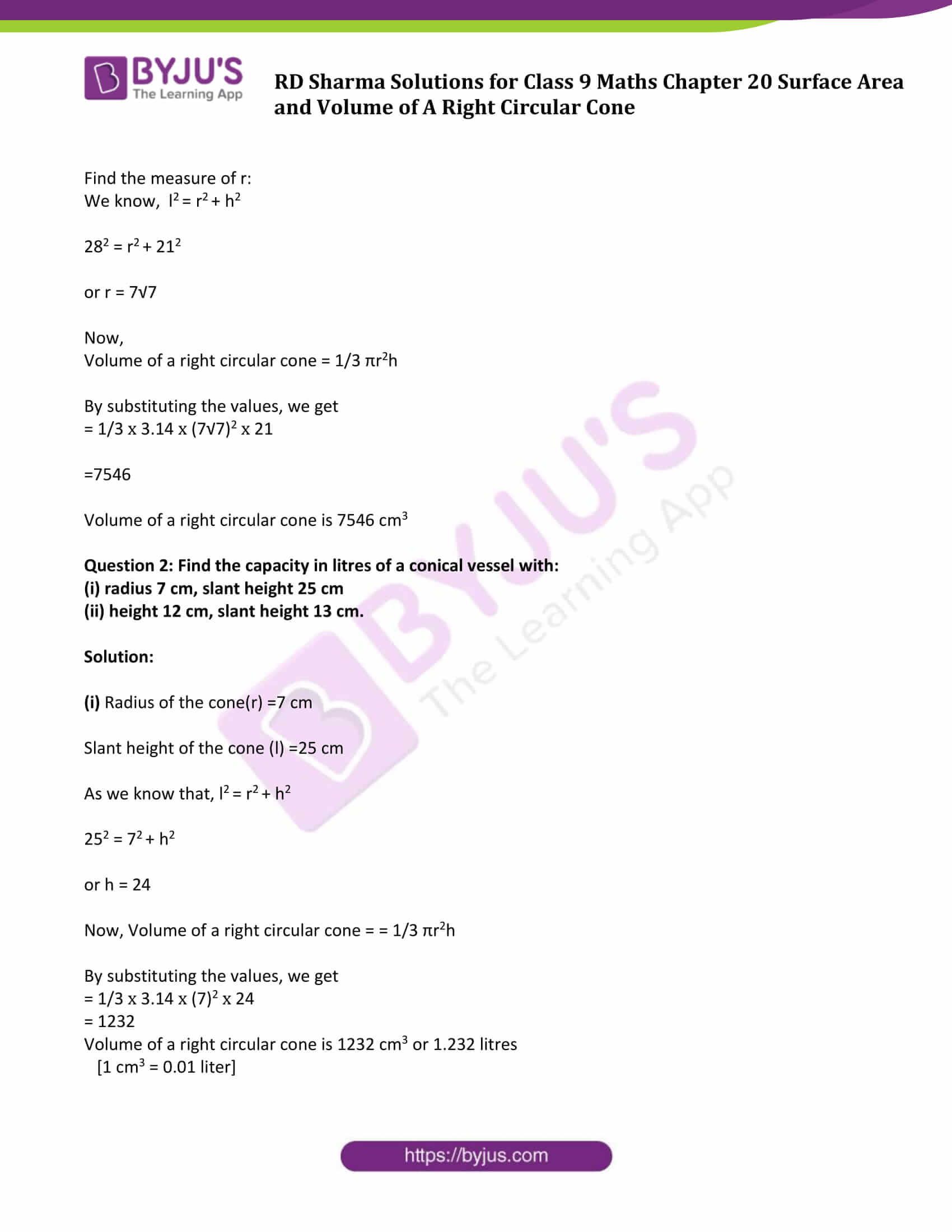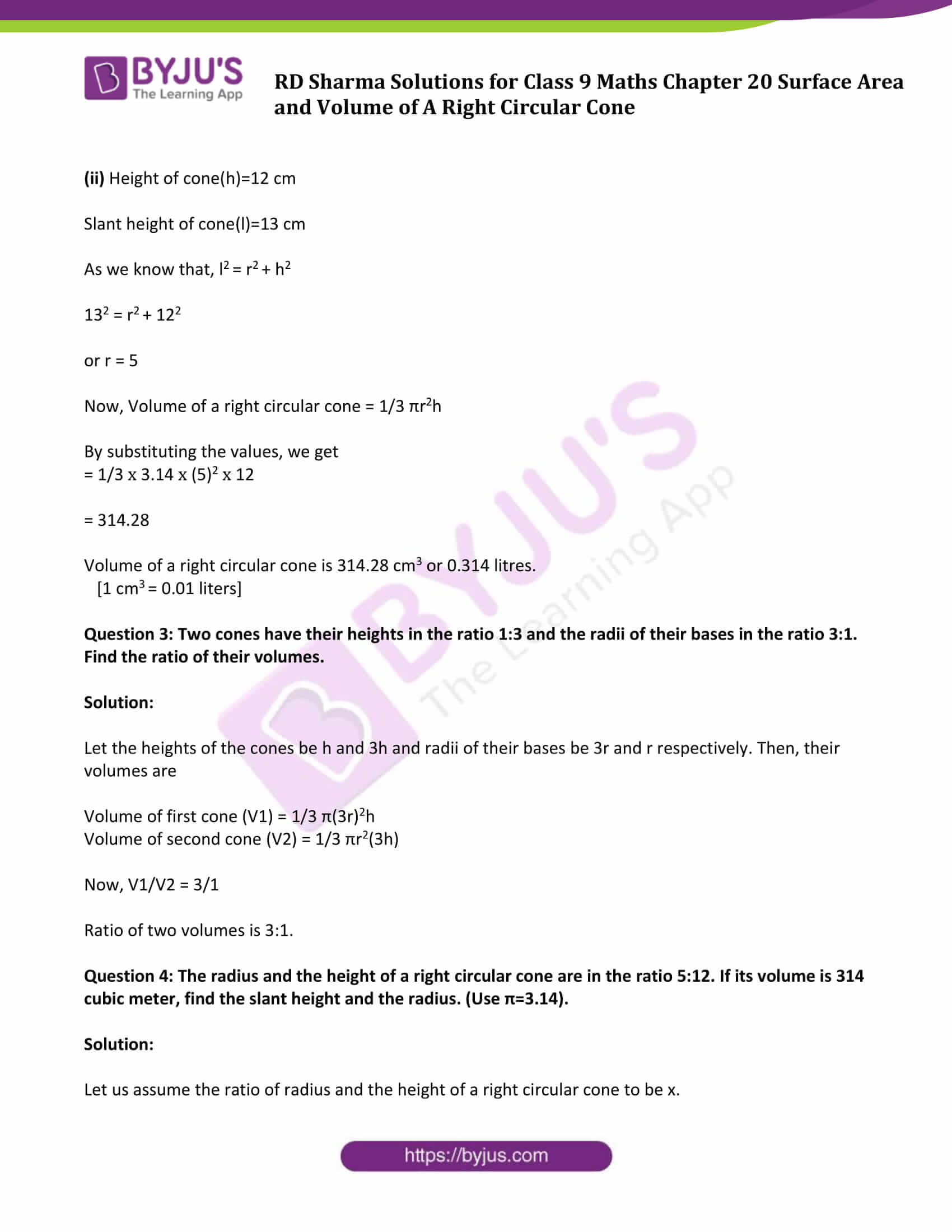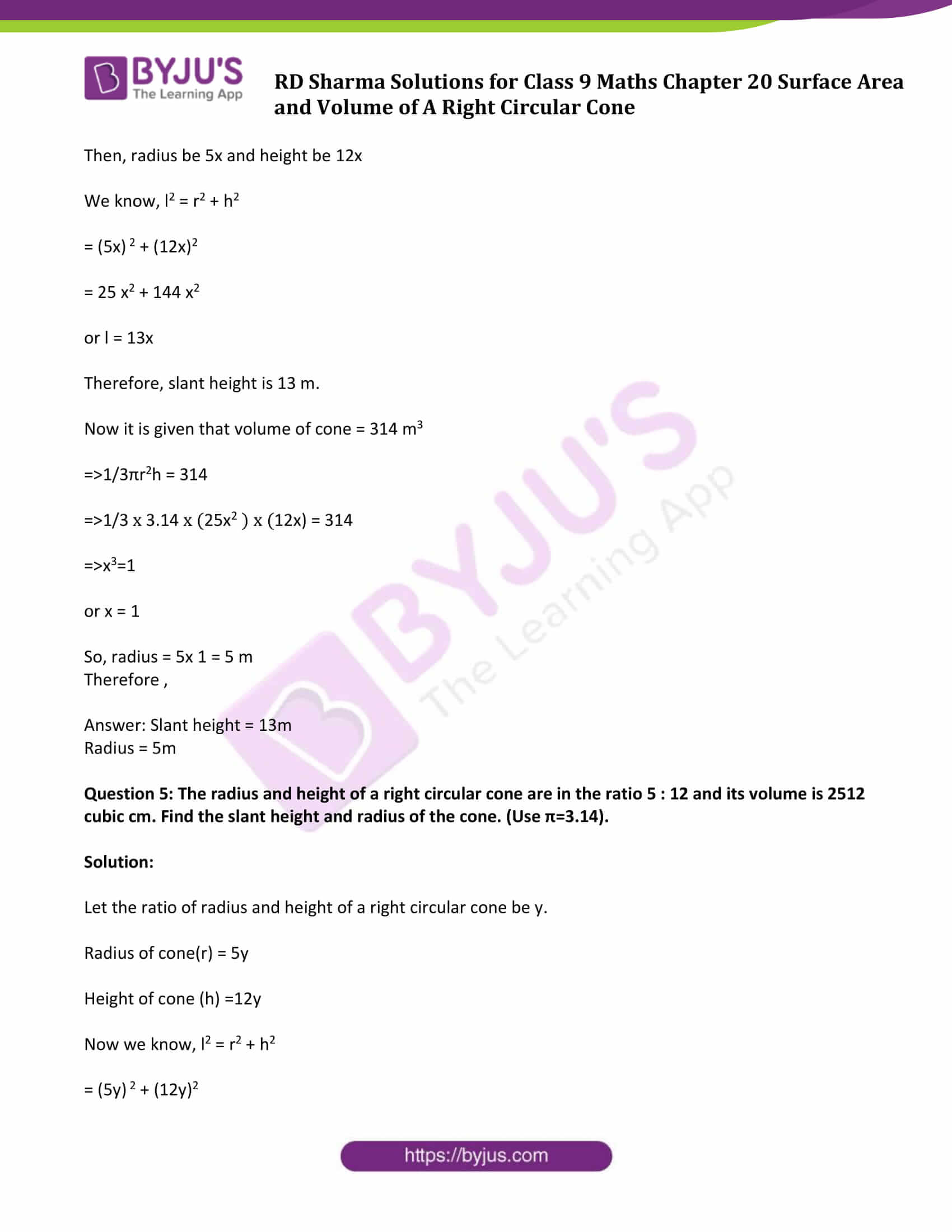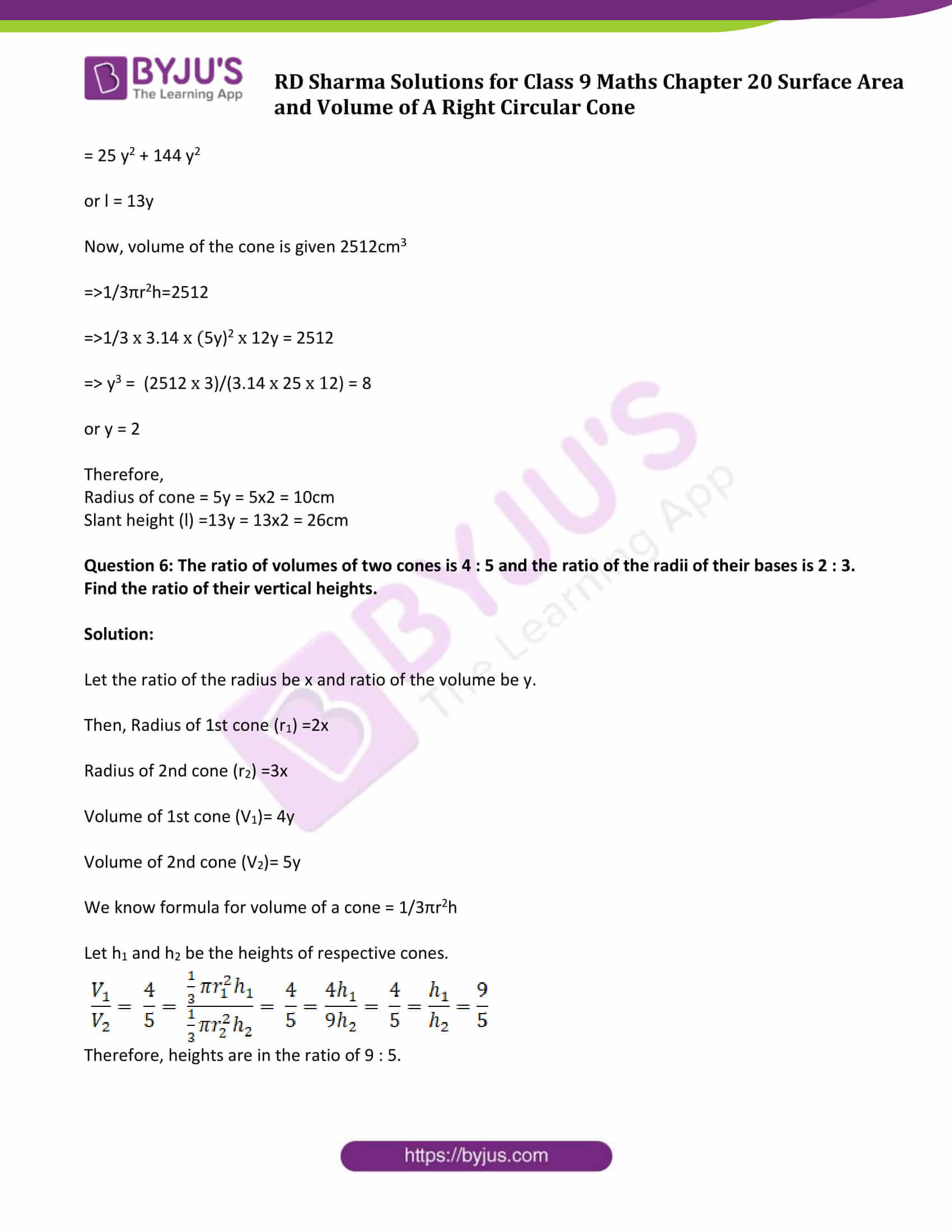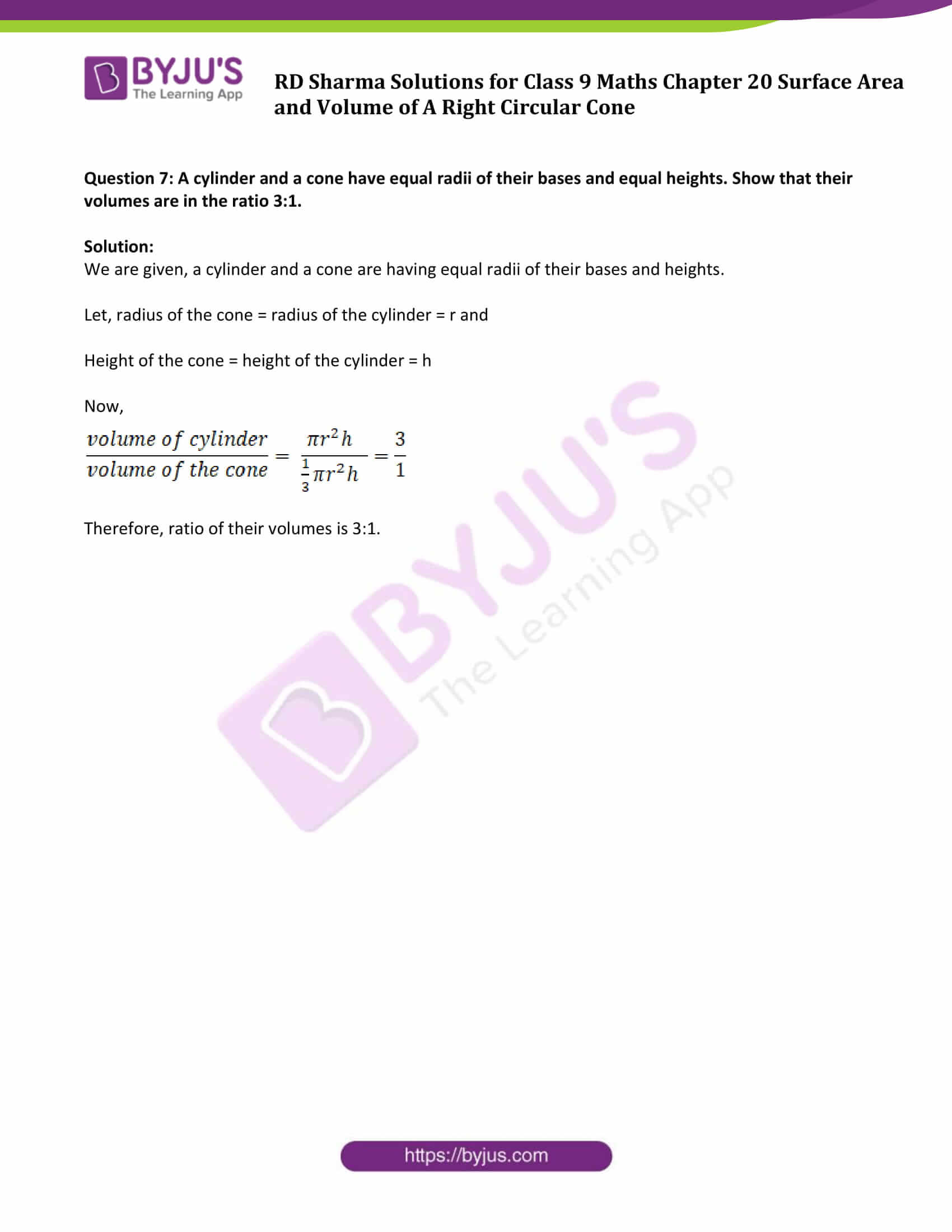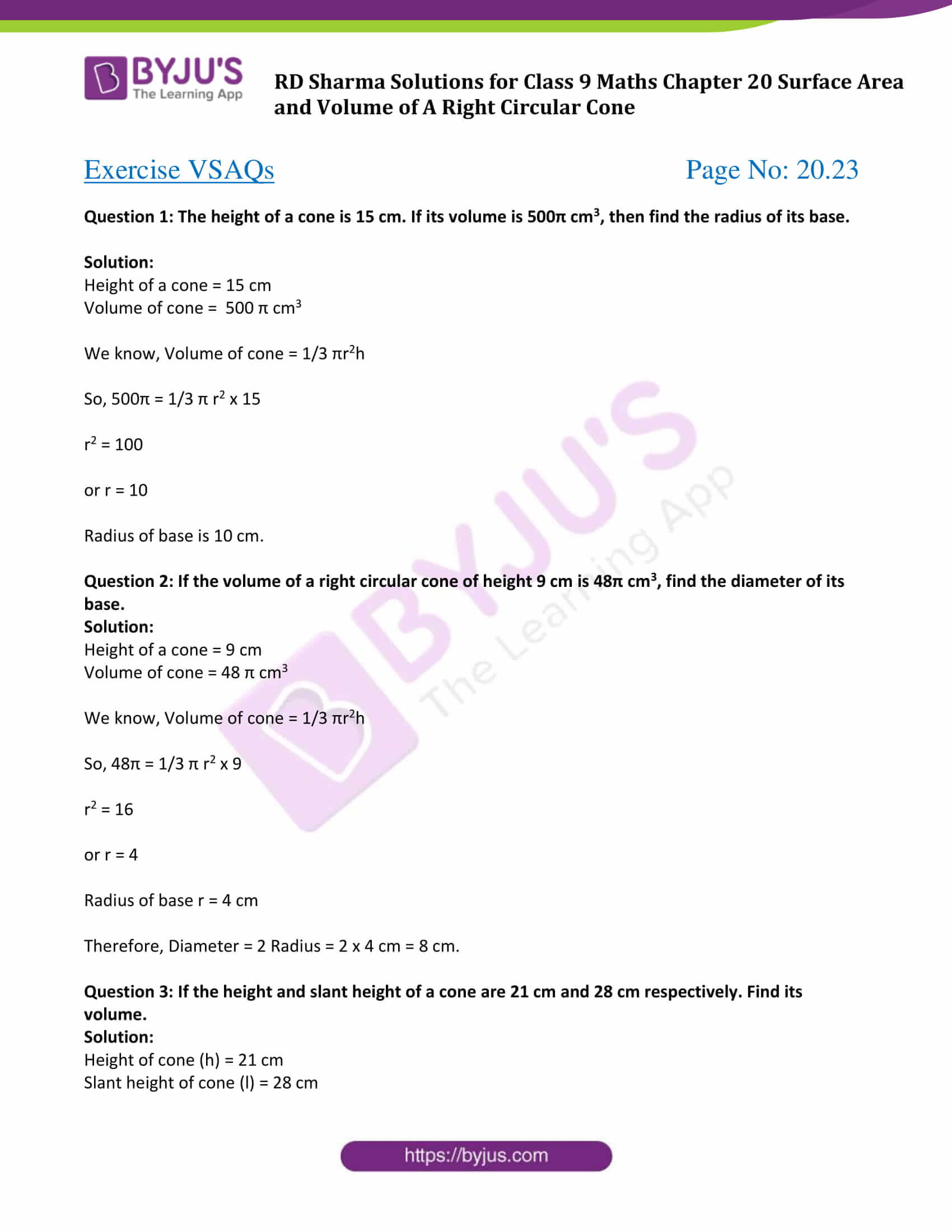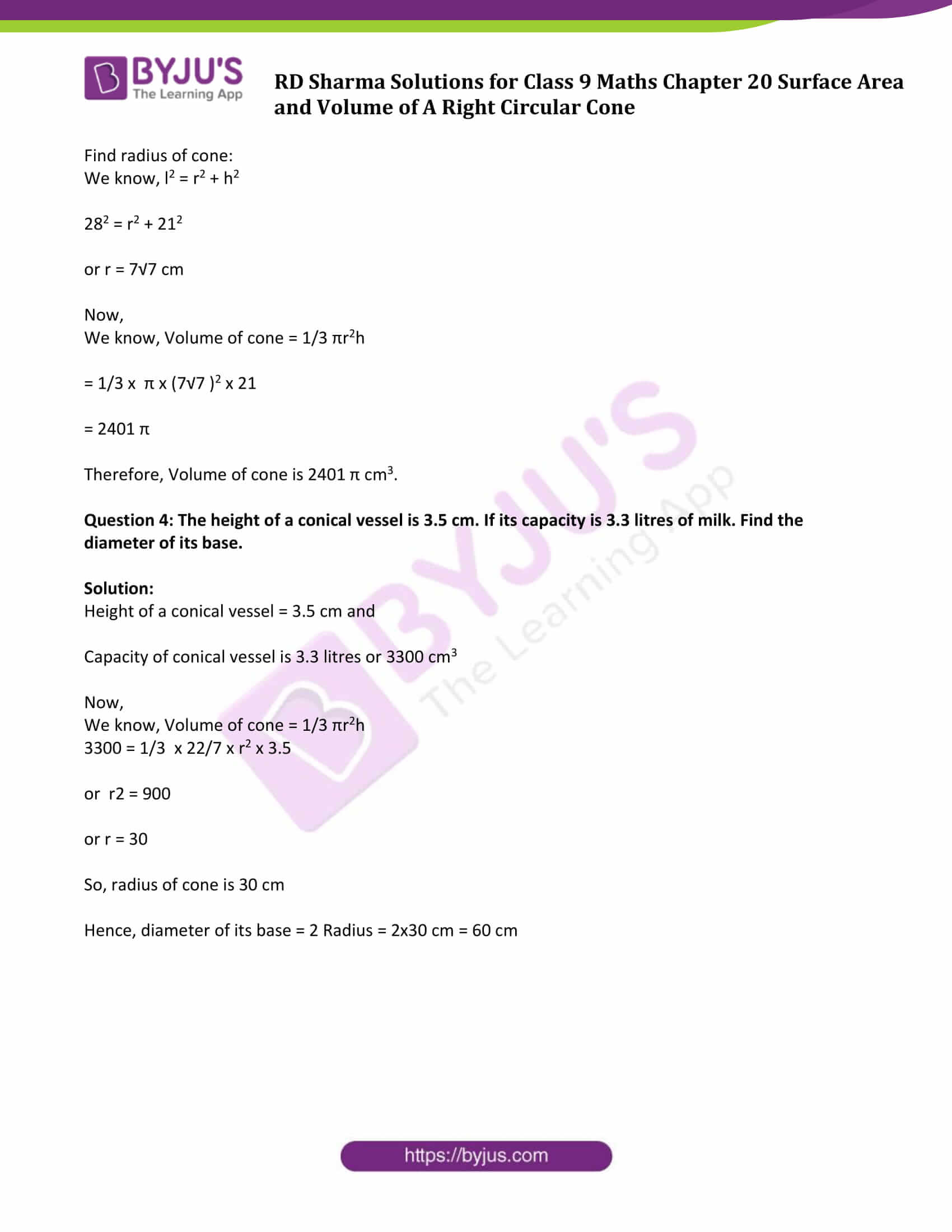### Exercise 20.1 Page No: 20.7

Question 1: Find the curved surface area of a cone, if its slant height is 60 cm and the radius of its base is 21 cm.

Solution:

Slant height of cone (l) = 60 cm

Radius of the base of the cone (r) = 21 cm

Now,

Curved surface area of the right circular cone = πrl = 22/7 x 21 x 60 = 3960 cm2

Therefore the curved surface area of the right circular cone is 3960 cm2

Question 2: The radius of a cone is 5cm and vertical height is 12cm. Find the area of the curved surface.

Solution:

Radius of cone (r) = 5 cm

Height of cone (h) = 12 cm

Find Slant Height of cone (l):

We know, l2 = r2 + h2

l2 = 52 +122

l2 = 25 + 144 = 169

Or l = 13 cm

Now,

C.S.A = πrl =3.14 x 5 x 13 = 204.28

Therefore, the curved surface area of the cone is 204.28 cm2

Question 3 : The radius of a cone is 7 cm and area of curved surface is 176 cm2 .Find the slant height.

Solution:

Radius of cone(r) = 7 cm

Curved surface area(C.S.A)= 176cm2

We know, C.S.A. = πrl

⇒ πrl = 176

⇒ 22/7 x 7 x l = 176

or l = 8

Therefore, slant height of the cone is 8 cm.

Question 4: The height of a cone 21 cm. Find the area of the base if the slant height is 28 cm.

Solution:

Height of cone(h) = 21 cm

Slant height of cone (l) = 28 cm

We know that, l2 = r2 + h2

282=r2+212

r2=282−212

or r= 7√7 cm

Now,

Area of the circular base = πr2

= 22/7 x (7√7 )2

=1078

Therefore, area of the base is 1078 cm2.

Question 5: Find the total surface area of a right circular cone with radius 6 cm and height 8 cm.

Solution:

Radius of cone (r) = 6 cm

Height of cone (h) = 8 cm

Total Surface area of the cone (T.S.A)=?

Find slant height of cone:

We know, l2 = r2 + h2

=62+82

= 36 + 64

= 100

or l = 10 cm

Now,

Total Surface area of the cone (T.S.A) = Curved surface area of cone + Area of circular base

= πrl + πr2

= (22/7 x 6 x 10) + (22/7 x 6 x 6)

= 1320/7 + 792/7

= 301.71

Therefore, area of the base is 301.71cm2.

Question 6: Find the curved surface area of a cone with base radius 5.25 cm and slant height 10 cm.

Solution:

Base radius of the cone(r) = 5.25 cm

Slant height of the cone(l) = 10 cm

Curved surface area (C.S.A) = πrl

=22/7 x 5.25 x 10

= 165

Therefore, curved surface area of the cone is 165cm2.

Question 7: Find the total surface area of a cone, if its slant height is 21 m and diameter of its base is 24 m.

Solution:

Diameter of the cone(d)=24 m

So, radius of the cone(r)= diameter/ 2 = 24/2 m = 12m

Slant height of the cone(l) = 21 m

T.S.A = Curved surface area of cone + Area of circular base

= πrl+ πr2

= (22/7 x 12 x 21) + (22/7 x 12 x 12)

= 1244.57

Therefore, total surface area of the cone is 1244.57 m2.

Question 8: The area of the curved surface of a cone is 60 π cm2. If the slant height of the cone be 8 cm, find the radius of the base.

Solution:

Curved surface area(C.S.A)= 60 π cm2

Slant height of the cone(l) = 8 cm

We know, Curved surface area(C.S.A )=πrl

⇒ πrl = 60 π

⇒ r x 8 = 60

or r = 60/8 = 7.5

Therefore, radius of the base of the cone is 7.5 cm.

Question 9: The curved surface area of a cone is 4070 cm2 and diameter is 70 cm .What is its slant height? (Use π =22/7)

Solution:

Diameter of the cone(d) = 70 cm

So, radius of the cone(r)= diameter/2 = 70/2 cm = 35 cm

Curved surface area = 4070 cm2

Now,

We know, Curved surface area = πrl

So, πrl = 4070

By substituting the values, we get

22/7 x 35 x l = 4070

or l = 37

Therefore, slant height of cone is 37 cm.

Question 10: The radius and slant height of a cone are in the ratio 4:7. If its curved surface area is 792 cm2, find its radius. (Use π =22/7)

Solution:

Curved surface area = 792 cm2

The radius and slant height of a cone are in the ratio 4:7 (Given)

Let 4x be the radius and 7x be the height of cone.

Now,

Curved surface area (C.S.A.) = πrl

So, 22/7 x (4x) x (7x) = 792

or x2 = 9

or x = 3

Therefore, Radius = 4x = 4(3) cm = 12 cm

### Exercise 20.2 Page No: 20.20

Question 1: Find the volume of the right circular cone with:

(iii) Height is 21cm and slant height 28cm

Solution:

Height of cone(h)=7cm

We know, Volume of a right circular cone = 1/3 πr2h

By substituting the values, we get

= 1/3 x 3.14 x 62 x 7

= 264

Volume of a right circular cone is 264 cm3

Height of cone(h)=12cm

Volume of a right circular cone = 1/3 πr2h

By substituting the values, we get

= 1/3 x 3.14 x 3.52 x 12

=154

Volume of a right circular cone is 154 cm3

(iii) Height of cone(h)=21 cm

Slant height of cone(l) = 28 cm

Find the measure of r:

We know, l2 = r2 + h2

282 = r2 + 212

or r = 7√7

Now,

Volume of a right circular cone = 1/3 πr2h

By substituting the values, we get

= 1/3 x 3.14 x (7√7)2 x 21

=7546

Volume of a right circular cone is 7546 cm3

Question 2: Find the capacity in litres of a conical vessel with:

(i) radius 7 cm, slant height 25 cm

(ii) height 12 cm, slant height 13 cm.

Solution:

(i) Radius of the cone(r) =7 cm

Slant height of the cone (l) =25 cm

As we know that, l2 = r2 + h2

252 = 72 + h2

or h = 24

Now, Volume of a right circular cone = = 1/3 πr2h

By substituting the values, we get

= 1/3 x 3.14 x (7)2 x 24

= 1232

Volume of a right circular cone is 1232 cm3 or 1.232 litres

[1 cm3 = 0.01 liter]

(ii) Height of cone(h)=12 cm

Slant height of cone(l)=13 cm

As we know that, l2 = r2 + h2

132 = r2 + 122

or r = 5

Now, Volume of a right circular cone = 1/3 πr2h

By substituting the values, we get

= 1/3 x 3.14 x (5)2 x 12

= 314.28

Volume of a right circular cone is 314.28 cm3 or 0.314 litres.

[1 cm3 = 0.01 liters]

Question 3: Two cones have their heights in the ratio 1:3 and the radii of their bases in the ratio 3:1. Find the ratio of their volumes.

Solution:

Let the heights of the cones be h and 3h and radii of their bases be 3r and r respectively. Then, their volumes are

Volume of first cone (V1) = 1/3 π(3r)2h

Volume of second cone (V2) = 1/3 πr2(3h)

Now, V1/V2 = 3/1

Ratio of two volumes is 3:1.

Question 4: The radius and the height of a right circular cone are in the ratio 5:12. If its volume is 314 cubic meter, find the slant height and the radius. (Use π=3.14).

Solution:

Let us assume the ratio of radius and the height of a right circular cone to be x.

Then, radius be 5x and height be 12x

We know, l2 = r2 + h2

= (5x) 2 + (12x)2

= 25 x2 + 144 x2

or l = 13x

Therefore, slant height is 13 m.

Now it is given that volume of cone = 314 m3

⇒1/3πr2h = 314

⇒1/3 x 3.14 x (25x2 ) x (12x) = 314

⇒x3=1

or x = 1

So, radius = 5x 1 = 5 m

Therefore ,

Question 5: The radius and height of a right circular cone are in the ratio 5 : 12 and its volume is 2512 cubic cm. Find the slant height and radius of the cone. (Use π=3.14).

Solution:

Let the ratio of radius and height of a right circular cone be y.

Height of cone (h) =12y

Now we know, l2 = r2 + h2

= (5y) 2 + (12y)2

= 25 y2 + 144 y2

or l = 13y

Now, volume of the cone is given 2512cm3

⇒1/3πr2h=2512

⇒1/3 x 3.14 x (5y)2 x 12y = 2512

⇒ y3 = (2512 x 3)/(3.14 x 25 x 12) = 8

or y = 2

Therefore,

Radius of cone = 5y = 5×2 = 10cm

Slant height (l) =13y = 13×2 = 26cm

Question 6: The ratio of volumes of two cones is 4 : 5 and the ratio of the radii of their bases is 2 : 3. Find the ratio of their vertical heights.

Solution:

Let the ratio of the radius be x and ratio of the volume be y.

Then, Radius of 1st cone (r1) =2x

Radius of 2nd cone (r2) =3x

Volume of 1st cone (V1)= 4y

Volume of 2nd cone (V2)= 5y

We know formula for volume of a cone = 1/3πr2h

Let h1 and h2 be the heights of respective cones.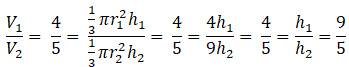Therefore, heights are in the ratio of 9 : 5.

Question 7: A cylinder and a cone have equal radii of their bases and equal heights. Show that their volumes are in the ratio 3:1.

Solution:

We are given, a cylinder and a cone are having equal radii of their bases and heights.

Let, radius of the cone = radius of the cylinder = r and

Height of the cone = height of the cylinder = h

Now,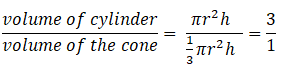Therefore, ratio of their volumes is 3:1.

### Exercise VSAQs Page No: 20.23

Question 1: The height of a cone is 15 cm. If its volume is 500π cm3, then find the radius of its base.

Solution:

Height of a cone = 15 cm

Volume of cone = 500 π cm3

We know, Volume of cone = 1/3 πr2h

So, 500π = 1/3 π r2 x 15

r2 = 100

or r = 10

Radius of base is 10 cm.

Question 2: If the volume of a right circular cone of height 9 cm is 48π cm3, find the diameter of its base.

Solution:

Height of a cone = 9 cm

Volume of cone = 48 π cm3

We know, Volume of cone = 1/3 πr2h

So, 48π = 1/3 π r2 x 9

r2 = 16

or r = 4

Radius of base r = 4 cm

Therefore, Diameter = 2 Radius = 2 x 4 cm = 8 cm.

Question 3: If the height and slant height of a cone are 21 cm and 28 cm respectively. Find its volume.

Solution:

Height of cone (h) = 21 cm

Slant height of cone (l) = 28 cm

We know, l2 = r2 + h2

282 = r2 + 212

or r = 7√7 cm

Now,

We know, Volume of cone = 1/3 πr2h

= 1/3 x π x (7√7 )2 x 21

= 2401 π

Therefore, Volume of cone is 2401 π cm3.

Question 4: The height of a conical vessel is 3.5 cm. If its capacity is 3.3 litres of milk. Find the diameter of its base.

Solution:

Height of a conical vessel = 3.5 cm and

Capacity of conical vessel is 3.3 litres or 3300 cm3

Now,

We know, Volume of cone = 1/3 πr2h

3300 = 1/3 x 22/7 x r2 x 3.5

or r2 = 900

or r = 30

So, radius of cone is 30 cm

Hence, diameter of its base = 2 Radius = 2×30 cm = 60 cm

### RD Sharma Solutions for Class 9 Maths Chapter 20 Surface Area and Volume of A Right Circular Cone

In the 20th Chapter of Class 9 RD Sharma Solutions students will study important concepts listed below:

• Right Circular Cone Introduction
• Some important terms definition – Vertex, Base, Axis, Radius and Slant Height.
• Surface Area of a Right Circular Cone
• Volume of a Right Circular Cone

Let us discuss some of the important terms used in this Chapter.

Total surface area: The total area occupied by the surface including the curved part and the base(s).

Curved surface area: The area occupied by the surface excluding the base(s) is known as curved surface area.

Volume: The space occupied by an object, which is measured in cubic units.

A right circular cone is a geometric figure having a circular base. Consider a right circular cone having ‘r’ as the base area, ‘h’ as the height of a cone and ‘l’ as a slant height. Then,

• Total surface area = πr(r + l),
• Curved surface area = πrl and
• Volume = 1/3πr2h

## Frequently Asked Questions on RD Sharma Solutions for Class 9 Maths Chapter 20

### Why should we follow RD Sharma Solutions for Class 9 Maths Chapter 20?

RD Sharma Solutions for Class 9 Maths Chapter 20 is the best reference material that offers complete and quality information about different Maths concepts. The questions given in the textbook have been solved in an easy-to-remember format, which further helps students to clearly understand and remember the answers. So, it’s clear that RD Sharma Textbooks for Class 9 are essential reference books to obtain a thorough knowledge of concepts. Practising these solutions on a regular basis is essential for in-depth exam preparation and score good marks in exams.

### How BYJU’S RD Sharma Solutions for Class 9 Maths Chapter 20 help the students in preparing for board exams?

Our solution module uses various examples and diagrams to explain the questions, wherever necessary. For CBSE students, practising RD Sharma Solutions for Class 9 Maths is essential to secure good marks in exams. These solutions curated by expert faculty in a simple language help students in gaining a better knowledge of the topics covered. Solving the questions from each exercise on a daily basis will ensure that students score high marks in the exams.

### What are the topics covered under RD Sharma Solutions for Class 9 Maths Chapter 20?

The topics covered in RD Sharma Solutions for Class 9 Maths Chapter 20 are as follows:
1. Right Circular Cone Introduction
2. Surface Area of a Right Circular Cone